# NMTA Middle Grades Math: Graphing Functions Chapter Exam

Exam Instructions:

Choose your answers to the questions and click 'Next' to see the next set of questions. You can skip questions if you would like and come back to them later with the yellow "Go To First Skipped Question" button. When you have completed the practice exam, a green submit button will appear. Click it to see your results. Good luck!

Answered 0 of 30

### Page 1

#### Question 3 3. Estimate the coordinates of the midpoint of the line segment below: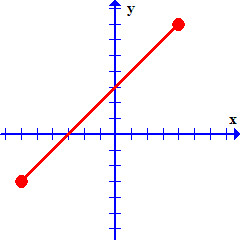### Page 2

#### Question 9 9. In the pictured graph of f(x) = -2x^2 + 4x - 2, for which values of x is the function decreasing?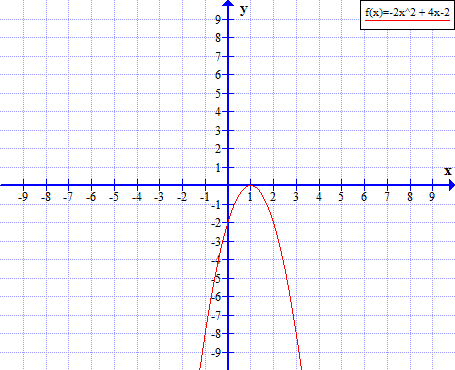### Page 4

#### Question 20 20. In the pictured graph of f(x) = x^2 + 6x, for which values of x is the function increasing?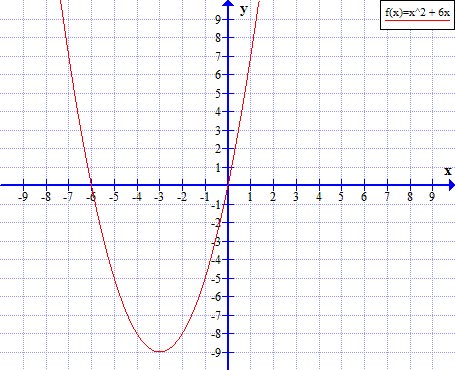### Page 5

#### Question 21 21. Which of the following shows the graph of the equation below?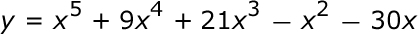#### Question 25 25. Which of the following shows the graph of the equation below?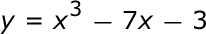### Page 6

#### Question 30 30. Which of the following shows the graph of the equation below?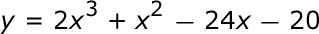#### NMTA Middle Grades Math: Graphing Functions Chapter Exam Instructions

Choose your answers to the questions and click 'Next' to see the next set of questions. You can skip questions if you would like and come back to them later with the yellow "Go To First Skipped Question" button. When you have completed the practice exam, a green submit button will appear. Click it to see your results. Good luck!

Support